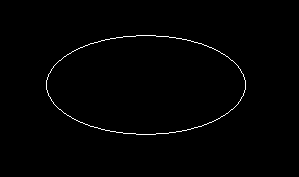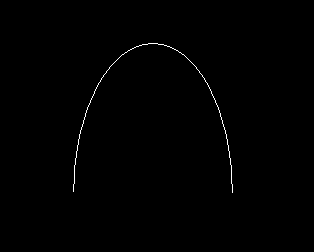# Draw ellipse in C graphics

Program to draw ellipse in C using graphics.h header file.
graphics.h library is used to include and facilitate graphical operations in program. C graphics using graphics.h functions can be used to draw different shapes, display text in different fonts, change colors and many more. Using functions of graphics.h you can make graphics programs, animations, projects and games. You can draw circles, lines, rectangles, bars and many other geometrical figures. You can change their colors using the available functions and fill them.
Examples:

```Input : x=250, y=200, start_angle = 0,
Output :Input : x=250, y=200, start_angle = 0,
Output :```

## Recommended: Please try your approach on {IDE} first, before moving on to the solution.

Explanation :The header file graphics.h contains ellipse() function which is described below :

void ellipse(int x, int y, int start_angle, int end_angle, int x_radius, int y_radius)

In this function x, y is the location of the ellipse. x_radius and y_radius decide the radius of form x and y.
start_angle is the starting point of angle and end_angle is the ending point of angle. The value of angle can vary from 0 to 360 degree.

 `// C Implementation for drawing ellipse ` `#include ` ` `  `int` `main() ` `{ ` `    ``// gm is Graphics mode which is a computer display ` `    ``// mode that generates image using pixels. ` `    ``// DETECT is a macro defined in "graphics.h" header file ` `    ``int` `gd = DETECT, gm; ` ` `  `    ``// location of ellipse ` `    ``int` `x = 250, y = 200; ` ` `  `    ``// here is the starting angle ` `    ``// and end angle ` `    ``int` `start_angle = 0; ` `    ``int` `end_angle = 360; ` ` `  `    ``// radius from x axis and y axis ` `    ``int` `x_rad = 100; ` `    ``int` `y_rad = 50; ` ` `  `    ``// initgraph initializes the graphics system ` `    ``// by loading a graphics driver from disk ` `    ``initgraph(&gd, &gm, ``""``); ` ` `  `    ``// ellipse function ` `    ``ellipse(x, y, start_angle, ` `     ``end_angle, x_rad, y_rad); ` ` `  `    ``getch(); ` ` `  `    ``// closegraph function closes the graphics ` `    ``// mode and deallocates all memory allocated ` `    ``// by graphics system . ` `    ``closegraph(); ` ` `  `    ``return` `0; ` `} `

Output:

``````

My Personal Notes arrow_drop_upCheck out this Author's contributed articles.

If you like GeeksforGeeks and would like to contribute, you can also write an article using contribute.geeksforgeeks.org or mail your article to contribute@geeksforgeeks.org. See your article appearing on the GeeksforGeeks main page and help other Geeks.

Please Improve this article if you find anything incorrect by clicking on the "Improve Article" button below.

Improved By : Akanksha_Rai

Article Tags :

Be the First to upvote.

Please write to us at contribute@geeksforgeeks.org to report any issue with the above content.